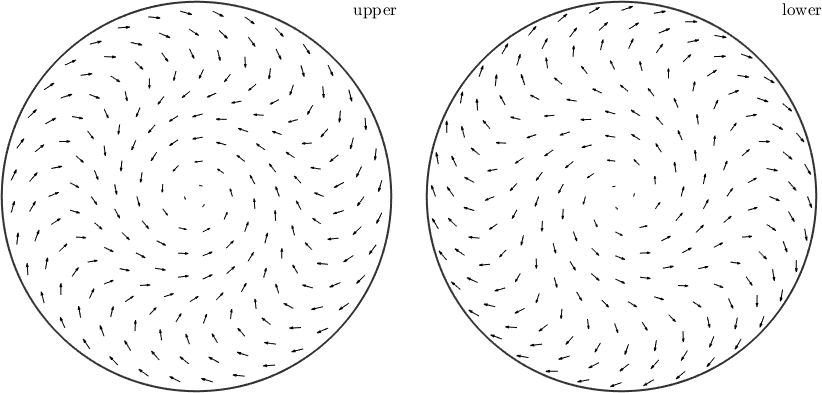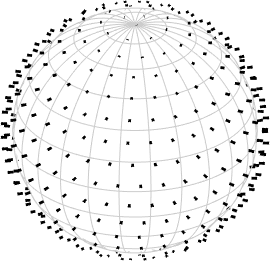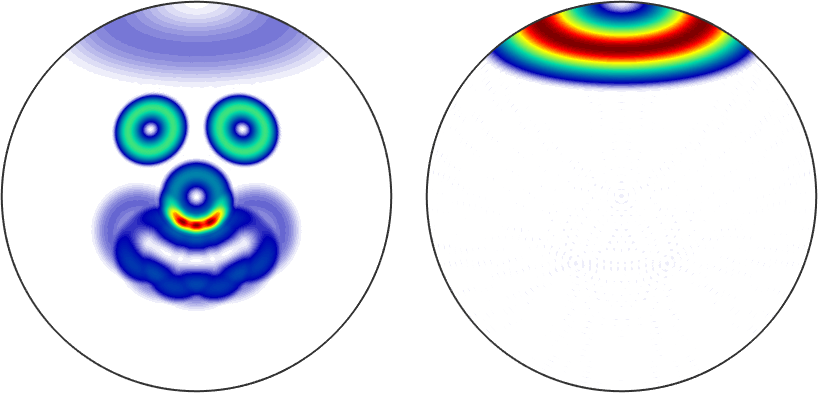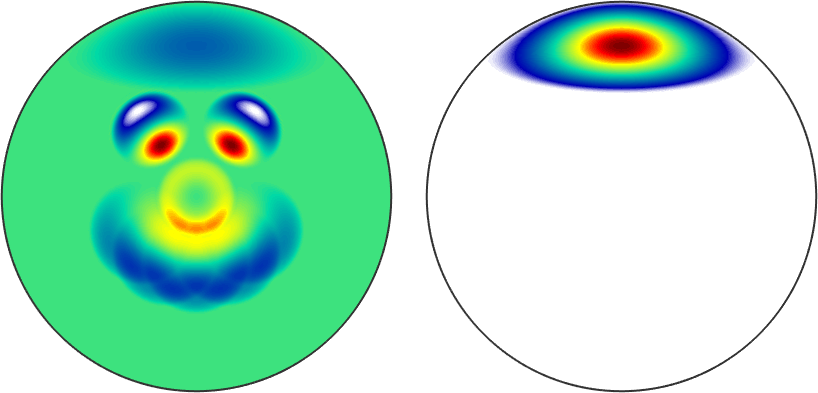S2VectorField edit page

S2VectorField handles three-dimensional functions on the sphere. For instance the gradient of an univariate S2FunHarmonic can return a S2VectorFieldHarmonic.

## Defining a S2VectorFieldHarmonic

Definition via function values

At first we need some example vertices

Next, we define function values for the vertices

Now the actual command to get sVF1 of type S2VectorFieldHarmonic

Definition via function handle

If we have a function handle for the function we could create a S2VectorFieldHarmonic via quadrature. At first lets define a function handle which takes vector3d as an argument and returns again vector3d:

Now we can call the quadrature command to get sVF2 of type S2VectorFieldHarmonic

Definition via S2FunHarmonic

If we directly call the constructor with a multivariate S2FunHarmonic with two or three entries it will create a S2VectorFieldHarmonic with sF(1) the polar angle and sF(2) the azimuth or sF(1), sF(2), and sF(3) the $$x$$, $$y$$, and $$z$$ component.

## Operations

Basic arithmetic operations

Again the basic mathematical operations are supported:

addition/subtraction of a vector field and a vector or addition/subtraction of two vector fields

multiplication/division by a scalar or a S2Fun

dot product with a vector or another vector field

cross product with a vector or another vector field

mean vector of the vector field

rotation of the vector field

pointwise norm of the vectors

## Visualization

One can use the default plot-command• same as quiver(sVF1)

or the 3D plot of a sphere with the vectors on itself## Some fun